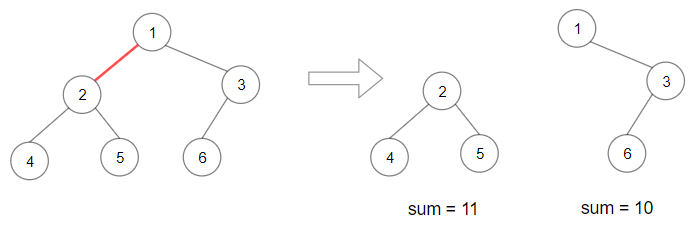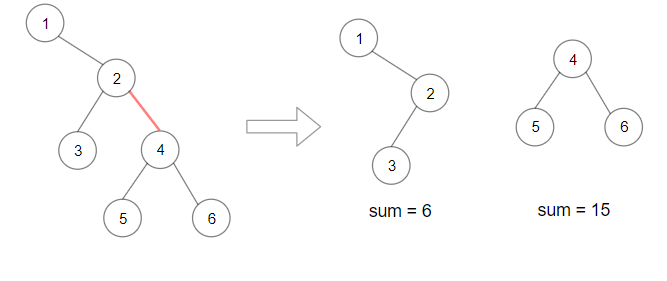Formatted question description: https://leetcode.ca/all/1339.html

# 1339. Maximum Product of Splitted Binary Tree (Medium)

Given a binary tree root. Split the binary tree into two subtrees by removing 1 edge such that the product of the sums of the subtrees are maximized.

Since the answer may be too large, return it modulo 10^9 + 7.

Example 1:Input: root = [1,2,3,4,5,6]
Output: 110
Explanation: Remove the red edge and get 2 binary trees with sum 11 and 10. Their product is 110 (11*10)


Example 2:Input: root = [1,null,2,3,4,null,null,5,6]
Output: 90
Explanation:  Remove the red edge and get 2 binary trees with sum 15 and 6.Their product is 90 (15*6)


Example 3:

Input: root = [2,3,9,10,7,8,6,5,4,11,1]
Output: 1025


Example 4:

Input: root = [1,1]
Output: 1


Constraints:

• Each tree has at most 50000 nodes and at least 2 nodes.
• Each node's value is between [1, 10000].

Related Topics:
Dynamic Programming, Tree

## Solution 1.

// OJ: https://leetcode.com/problems/maximum-product-of-splitted-binary-tree/

// Time: O(N)
// Space: O(H)
class Solution {
long long s = 0, mod = 1e9 + 7, ans = 0;
int postorder(TreeNode *root) {
if (!root) return 0;
int sum = root->val + postorder(root->left) + postorder(root->right);
if (s) ans = max(ans, sum * (s - sum));
return sum;
}
public:
int maxProduct(TreeNode* root) {
s = postorder(root);
postorder(root);
return ans % mod;
}
};


Java

/**
* Definition for a binary tree node.
* public class TreeNode {
*     int val;
*     TreeNode left;
*     TreeNode right;
*     TreeNode(int x) { val = x; }
* }
*/
class Solution {
public int maxProduct(TreeNode root) {
final int MODULO = 1000000007;
List<TreeNode> nodesList = new ArrayList<TreeNode>();
Map<TreeNode, TreeNode> childParentMap = new HashMap<TreeNode, TreeNode>();
queue.offer(root);
while (!queue.isEmpty()) {
TreeNode node = queue.poll();
TreeNode left = node.left, right = node.right;
if (left != null) {
childParentMap.put(left, node);
queue.offer(left);
}
if (right != null) {
childParentMap.put(right, node);
queue.offer(right);
}
}
for (int i = nodesList.size() - 1; i > 0; i--) {
TreeNode node = nodesList.get(i);
TreeNode parent = childParentMap.get(node);
if (parent != null)
parent.val += node.val;
}
long maxProduct = 0;
for (int i = nodesList.size() - 1; i > 0; i--) {
TreeNode node = nodesList.get(i);
int value1 = node.val, value2 = root.val - node.val;
long product = (long) value1 * (long) value2;
maxProduct = Math.max(maxProduct, product);
}
int maxProductModulo = (int) (maxProduct % MODULO);
return maxProductModulo;
}
}